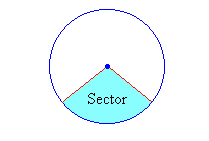## How to Calculate and Solve for the Perimeter or Circumference, Diameter, Radius and Angle of a Sector | The Calculator EncyclopediaThe image above is a sector.

To compute the Perimeter or Circumference a sector, two essential parameters is needed and this parameters are the radius of the sector (r) and the angle of the sector (θ). You can also use the diameter of the sector (d).

The formula for calculating the Perimeter or Circumference of a sector:

P = 2r + θ / 360(2πr)

Where;

P = Perimeter or Circumference
r = Radius of the sector
θ = Angle of the Sector

Let’s solve an example:
Find the perimeter or circumference of a sector when the radius of the sector is 14 cm and the angle of the sector is 60°

This implies that;

r = Radius of the sector = 14 cm
θ = Angle of the sector = 60°

P = 2r + θ / 360(2πr)
P = 2 x 14 + 60 / 360(2 x 3.142 x 14)
P = 28 + 0.1667 (87.97)
P = 28 + 14.66
P = 42.66

Therefore, the perimeter or circumference of the sector is 42.66 cm.

Calculating the Perimeter or Circumference of a Sector using Diameter and Angle of the sector.

P = d + θ / 360(πd)

θ = Angle of the sector
d = Diameter of the sector

Let’s solve an example;
Find the perimeter or circumference of a sector when the diameter of the sector is 20 cm and the angle of the sector is 80°.

This implies that;

d = Diameter of the sector = 20 cm
θ = Angle of the sector = 80°